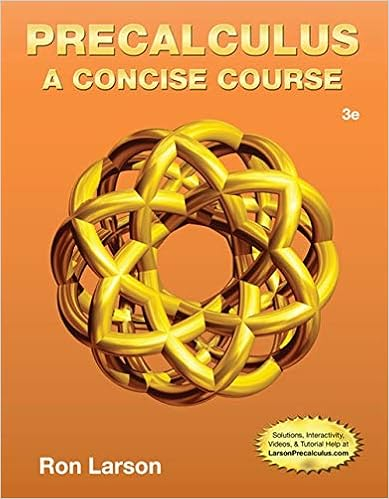# Evaluate the indefinite integral as an infinite

• Homework Help
• gatorguy803
• 12

This preview shows page 8 - 10 out of 12 pages.

##### We have textbook solutions for you!
The document you are viewing contains questions related to this textbook.The document you are viewing contains questions related to this textbook.
Chapter 1 / Exercise 67
Precalculus: A Concise Course
LarsonExpert Verified
Evaluate the indefinite integral as an infinite series: arctan( x 2 ) dx . 203. Use series to approximate the definite integral correct to three decimal places: 1 0 x cos( x 5 ) dx . 204. Use series to evaluate the limit: lim x 0 5 x - arctan(5 x ) x 3 . Curves Defined by Parametric Equations Directions: Eliminate the parameter to find a Cartesian equation of the curve. 205. x = e t - 6, y = e 2 t 206. x = ln t , y = t , t 36 Directions: Determine what curve is represented by the parametric equations. Be sure to indicate direction as well as any starting or ending points.
##### We have textbook solutions for you!
The document you are viewing contains questions related to this textbook.The document you are viewing contains questions related to this textbook.
Chapter 1 / Exercise 67
Precalculus: A Concise Course
LarsonExpert Verified
207. x = 2 cos(3 t ), y = 2 sin(3 t ), 0 t 2 π / 3 208. x = sin(4 t ), y = sin 2 (4 t ) Calculus with Parametric Curves Directions: Answer the questions. 209. Find an equation of the tangent to x = t 4 + 2, y = t 3 + t at the point corresponding to t = 1. 210. Find an equation of the tangent to x = 2 + ln t , y = t 2 + 2 at the point (2 , 3) by both eliminating the parameter and without eliminating the parameter. 211. Consider x = 3 + t 2 , y = t 2 + t 3 . (a) Find dy dx and d 2 y dx 2 . (b) For which values of t is the curve concave upward? 212. Consider x = 10 - t 2 , y = t 3 - 27 t . (a) Find the points on the curve where the tangent is horizontal. (b) Find the points on the curve where the tangent is vertical. 213. Consider x = 3 cos θ , y = sin 2 θ . (a) Find the points on the curve where the tangent is horizontal. (a) Find polar coordinates ( r, θ ) of the point (2 , - 2), where r > 0 and 0 θ 2 π . (b) Find polar coordinates ( r, θ ) of the point (2 , - 2), where r < 0 and 0 θ 2 π . (c) Find polar coordinates ( r, θ ) of the point (1 , 3), where r > 0 and 0 θ 2 π . (d) Find polar coordinates ( r, θ ) of the point (1 , 3), where r < 0 and 0 θ 2 π . 219. Find a Cartesian equation for the polar curve r = 6 sin θ and identify the curve. Directions: Sketch the graph of the given polar equation. 220. r = sin θ 221. r = - 7 cos θ 222. r = 5(1 - sin θ ), θ 0 Polar Coordinates Directions: Answer the questions. 218. The Cartesian coordinates of a point are given.
(b) Find the points on the curve where the tangent is vertical. 214. Consider x = 5 cos t , y = 2 sin t cos t . Show
that this curve has two tangents at (0 , 0) and find their equations. 215. Consider x = t - t 2 , y = 4 t 3 / 2 / 3,
3 t 5. Setup an integral that represents the length of the curve and then evaluate that integral. 216. Find the length of the curve: x = 1 + 3 t 2 ,
y = 9 + 2 t 3 , 0 t 3.
217. Find the length of the curve: x = e t + e - t , y = 5 - 2 t , 0 t 3.
•••